## Practical Course: SMT solving for real arithmetic with simplex

Satisfiability Checking is the task of checking the existence of a satisfying solution for a logical formula. For propositional logic formulas, being Boolean combinations of propositions such as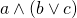, SAT solvers can be used to determine whether there is an assignment mapping to each proposition a value either true or false, such that substituting these values into the formula, the formula evaluates to true.

For the satisfiability check of quantifier-free first-order logic formulas over different theories, SAT solvers can be extended with theory solver modules, resulting in SAT-modulo-theories (SMT) solvers. Thereby we move from checking satisfiability for propositional logic formulas, such as, towards checking satisfiability for formulas such as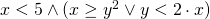. Such logics can be syntactically more rich as well as semantically more expressive than propositional logic, and allow the natural formalisation of a wide range of practical problems, e.g., in scheduling, planning, software verification, or controller synthesis.

This practical course aims at the design, implementation, and optimization of state-of-the-art decision procedures for SMT solving for the theory of linear real arithmetic. This theory allows for arbitrary equalities and inequalities over linear expressions containing real variables, for example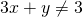or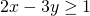. The most notable restriction is that nonlinear expressions are forbidden, meaning that no multiplication is allowed between variables like in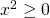or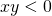.

A well-known approach for this theory is the Simplex algorithm that is in widespread use in virtually all solvers for linear real arithmetic. We will start with the implementation of a basic simplex algorithm and continue with some extensions or optimizations, for example an optimized internal datastructure, better support for incrementality or make it applicable it to linear integer problems.

The implementation will take place within the SMT-RAT project and can benefit from its manifold features. The resulting SMT solver will be tested on the official SMT-LIB benchmarks and be compared to state-of-the-art SMT solvers. Furthermore, we will form at least two teams and compare their solvers within a competition.